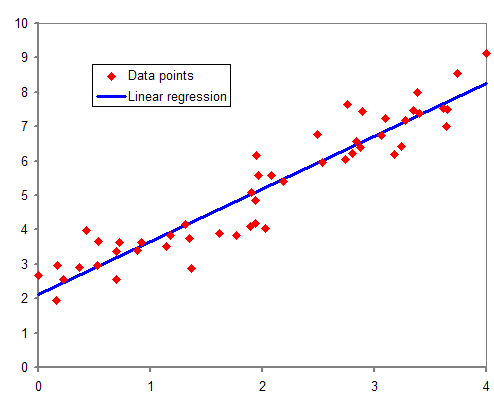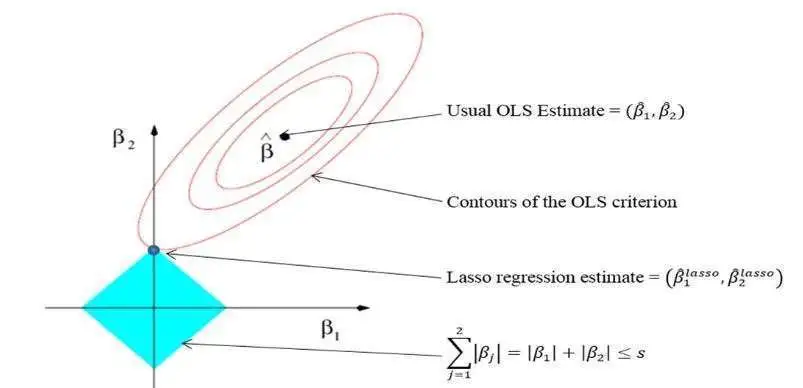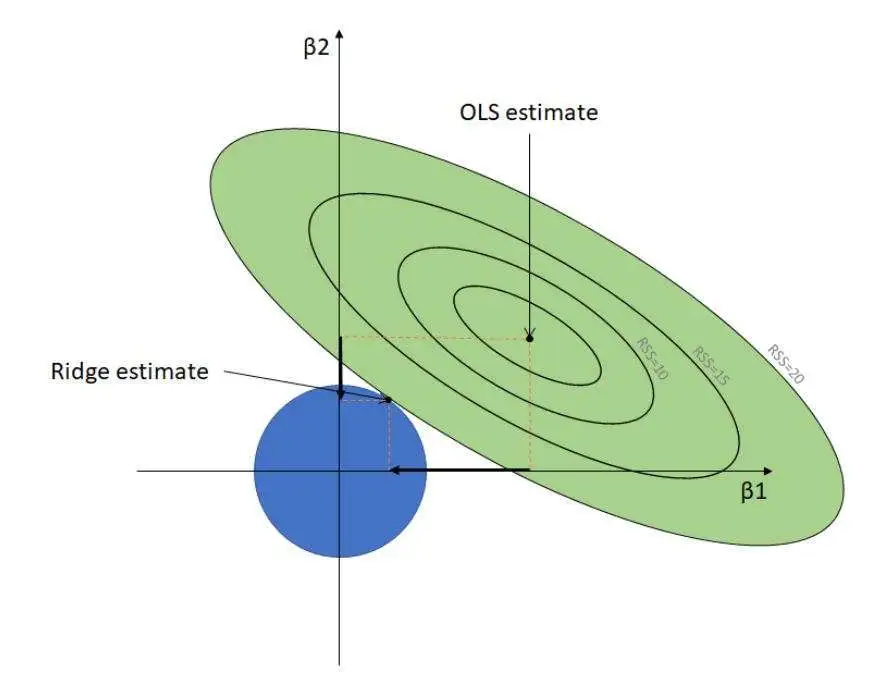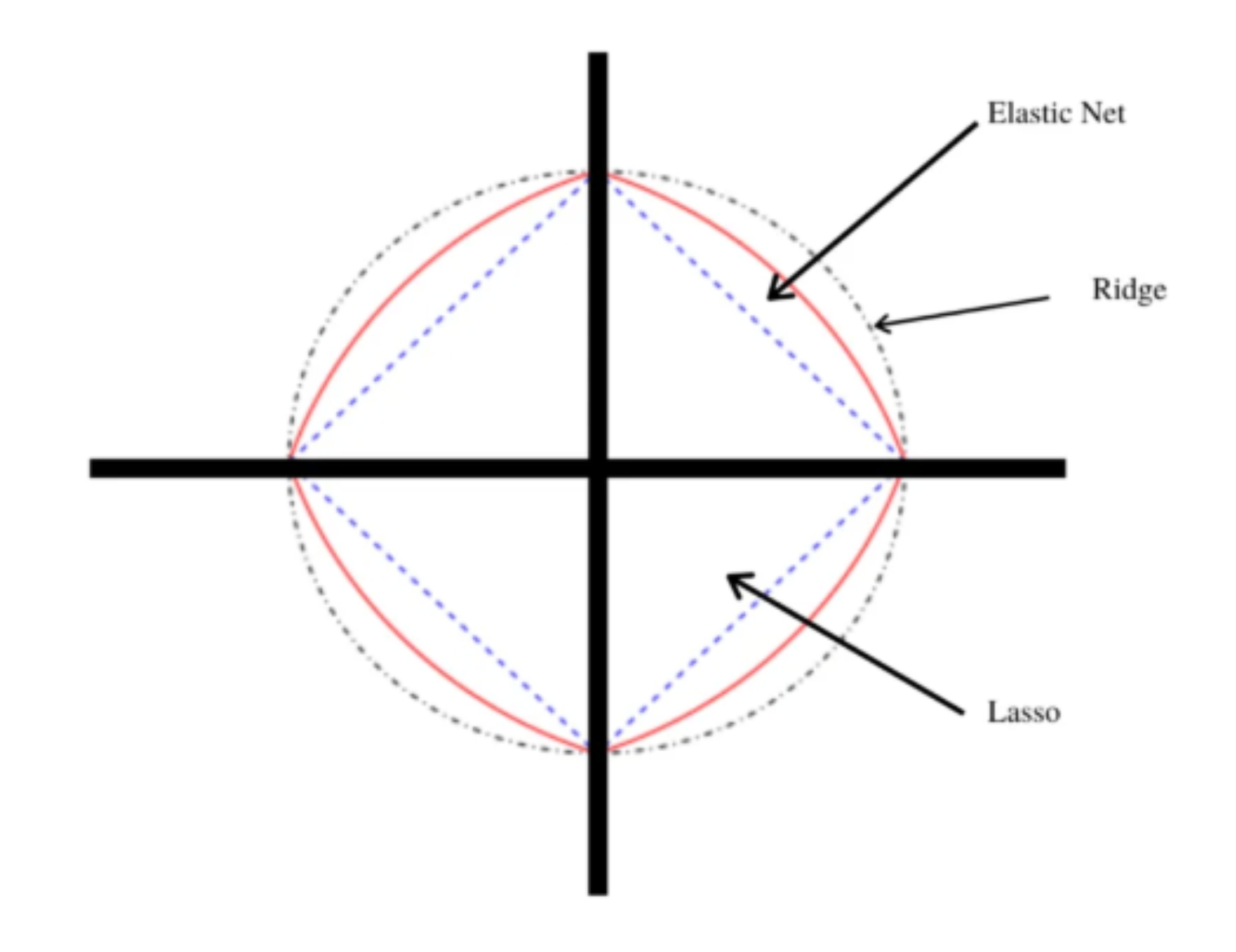# 什么是机器学习中的回归算法？scikit-learn中的四大回归算法学习

2023-01-23 18:27:40  阅读：449  来源： 互联网

## 1. 线性回归``y=mx+c+e``

``````# Import libraries
import numpy as np
from sklearn.linear_model import LinearRegression
# Prepare input data
# X represents independent variables
X = np.array([[1, 1], [1, 2], [1, 3], [2, 1], [2, 2], [2, 3]])
# Regression equation: y = 1 * x_0 + 2 * x_1 + 3
# y represents dependant variable
y = np.dot(X, np.array([1, 2])) + 3
# array([ 6,  8, 10,  7,  9, 11])
reg = LinearRegression().fit(X, y)
reg.score(X, y)
# Regression coefficients
reg.coef_
# array([1., 2.])
reg.intercept_
# 2.999999999999999
reg.predict(np.array([[4, 4]]))
# array([15.])
reg.predict(np.array([[6, 7]]))
array([23.])``````

## 2. Lasso回归——最小绝对收缩和选择算子LASSO 回归是线性回归的扩展，它在模型训练期间向损失函数添加惩罚（L1）以限制（或缩小）回归系数的值。此过程称为 L1 正则化。

L1 正则化缩小了对预测任务没有显着贡献的输入特征的回归系数值。它将这些系数的值降为零，并从回归方程中删除相应的输入变量，从而鼓励使用更简单的回归模型。

``````# Import library
from sklearn import linear_model
# Building lasso regression model with hyperparameter alpha = 0.1
clf = linear_model.Lasso(alpha=0.1)
# Prepare input data
X = np.array([[1, 1], [1, 2], [1, 3], [2, 1], [2, 2], [2, 3]])
y = [ 6,  8, 10,  7,  9, 11]
clf.fit([[0,0], [1, 1], [2, 2]], [0, 1, 2])
# Regression coefficients
clf.coef_
# array([0.6 , 1.85])
clf.intercept_
# 3.8999999999999995
clf.predict(np.array([[4, 4]]))
# array([13.7])
clf.predict(np.array([[6, 7]]))
# array([20.45])``````

## 3.岭回归算法L2 正则化通过最小化此类自变量的影响来处理多重共线性，将相应回归系数的值降低到接近于零。与 L1 正则化不同，它防止完全删除任何变量。

``````# Import library
from sklearn.linear_model import Ridge
# Building ridge regression model with hyperparameter alpha = 0.1
clf = Ridge(alpha=0.1)
# Prepare input data
X = np.array([[1, 1], [1, 2], [1, 3], [2, 1], [2, 2], [2, 3]])
y = [ 6,  8, 10,  7,  9, 11]
clf.fit(X, y)
clf.coef_
# array([0.9375, 1.95121951])
clf.intercept_
# 3.1913109756097553
clf.predict(np.array([[4, 4]]))
# array([14.74618902])
clf.predict(np.array([[6, 7]]))
# array([22.47484756])``````

## 4. 弹性网络回归算法弹性网络回归是一种正则化线性回归算法，在训练过程中将 L1 和 L2 惩罚项线性组合并添加到损失函数中。它通过为每个惩罚分配适当的权重来平衡套索回归和岭回归，从而提高模型性能。

Elastic net 有两个可调超参数，即 alpha 和 lambda。Alpha 确定赋予每个惩罚的权重百分比，而 lambda 控制对模型性能有贡献的两种负债的加权和的百分比。

``````# Import library
from sklearn.linear_model import ElasticNet
# Building elastic net regression model with hyperparameter alpha = 0.1
regr = ElasticNet(alpha=0.1)
# Prepare input data
X = np.array([[1, 1], [1, 2], [1, 3], [2, 1], [2, 2], [2, 3]])
y = [ 6,  8, 10,  7,  9, 11]
regr.fit(X, y)
regr.coef_
# array([0.66666667, 1.79069767])
regr.intercept_)
# 3.9186046511627914
regr.predict([[4, 4]])
# array([13.74806202])
regr.predict([[0, 0]])
# array([20.45348837])``````

## 回归可以处理线性依赖

2. 关于本站的所有留言、评论、转载及引用，纯属内容发起人的个人观点，与本站观点和立场无关；
3. 关于本站的所有言论和文字，纯属内容发起人的个人观点，与本站观点和立场无关；
4. 本站文章均是网友提供，不完全保证技术分享内容的完整性、准确性、时效性、风险性和版权归属；如您发现该文章侵犯了您的权益，可联系我们第一时间进行删除；
5. 本站为非盈利性的个人网站，所有内容不会用来进行牟利，也不会利用任何形式的广告来间接获益，纯粹是为了广大技术爱好者提供技术内容和技术思想的分享性交流网站。